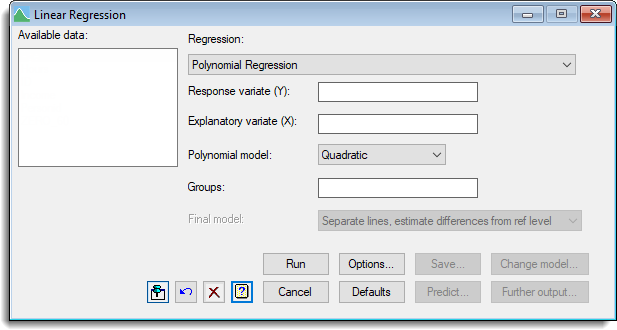1. Home
2. Polynomial Regression

# Polynomial Regression

Select menu: Stats | Regression Analysis | Linear Models

You can use the Polynomial regression downdown list option to fit polynomials representing quadratic, cubic or quartic curves.## Response variate

Specifies the name of the response (or y-) variate.

## Explanatory variate

Specifies the name of the explanatory (or x-) variate.

## Polynomial model

Provides a list of polynomial models than can be fitted. You can choose either Linear, Quadratic, Cubic or Quartic.

## Groups

If the data values are classified into groups you can supply a factor defining the different categories. When a grouping factor is supplied a series of models are fitted.

The first model fitted is a polynomial regression, ignoring the groups. Next the model is extended to include a different constant (or intercept) for each group, giving a set of parallel lines one for each group. Then, the final model has both a different constant and a different regression coefficient (or slope) for each group. The list adjacent to the Groups box field you select between the types of regression model that you want to fit.

## Final model

For an analysis of parallelism, if the analysis shows that different intercepts are needed but not different slopes, you can use this option to select the final model and re-run the analysis to remove the interaction between the polynomial and the groups factor. Similarly, if different intercepts are not needed this option can be used to fit just the polynomial model.